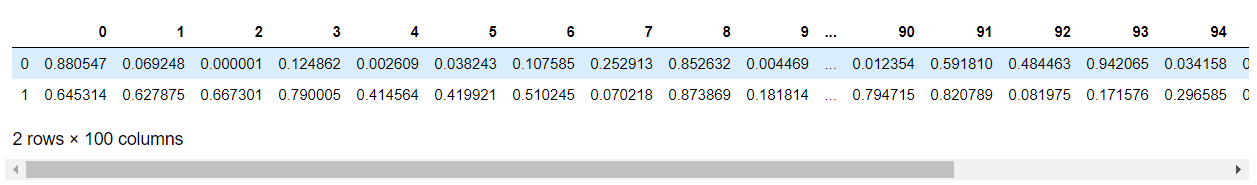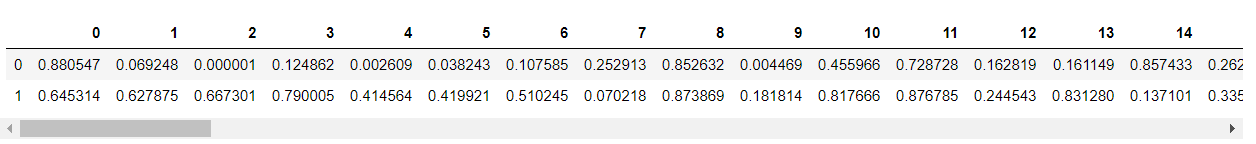# How to widen output display to see more columns in Pandas dataframe?

In Python, if there are many more number of columns in the dataframe, then not all the columns will be shown in the output display. So, let’s see how to widen output display to see more columns.

Method 1: Using pandas.set_option() function.

This function is used to set the value of a specified option.

Syntax: pandas.set_option(pat, value)

Returns: None

Example:

## Python3

 `# importing numpy library  ` `import` `numpy as np ` ` `  `# importing pandas library ` `import` `pandas as pd ` ` `  `# define a dataframe of ` `# 2 rows and 100 columns ` `# with random entries ` `df ``=` `pd.DataFrame(np.random.random(``200``).reshape(``2``, ``100``)) ` ` `  `# show the dataframe ` `df `

Output:To print the above output in a wider format using pandas.set_option() function.

## Python3

 `# using pd.set_option() ` `# to widen the output  ` `# display ` `pd.set_option(``'display.max_columns'``, ``100``) ` ` `  `# show the dataframe ` `df `

Output:Method 2: Using pd.options.display.max_columns attribute.

This attribute is used to set the no. of columns to show in the display of the pandas dataframe.

Example:

## Python3

 `# importing numpy library ` `import` `numpy as np ` ` `  `# importing pandas library ` `import` `pandas as pd ` ` `  `# define a dataframe of ` `# 15 rows and 200 columns ` `# with random entries ` `df ``=` `pd.DataFrame(np.random.randint(``0``, ``100``,  ` `                                    ``size ``=``(``15``, ``200``))) ` ` `  `# show the dataframe ` `df `

Output:To print the above output in a wider format using pd.options.display.max_columns attribute.

## Python3

 `# using an alternative ` `# way to use  ` `# pd.set_option() method ` `# to widen the output  ` `# display ` `pd.options.display.max_columns ``=` `200` ` `  `# show the dataframe ` `df `

Output:Whether you're preparing for your first job interview or aiming to upskill in this ever-evolving tech landscape, GeeksforGeeks Courses are your key to success. We provide top-quality content at affordable prices, all geared towards accelerating your growth in a time-bound manner. Join the millions we've already empowered, and we're here to do the same for you. Don't miss out - check it out now!

Previous
Next